# What is a Transversal Line? - Angles & Examples

Dawn Wells, Cathryn Jackson
• Author
Dawn Wells

Dawn has over 14 years of math teaching and tutoring experience covering middle school, high school and dual enrollment classes. She has taught middle school math, Algebra, Geometry, Algebra II, college Algebra and Trigonometry. She has a Master's degree in Innovative Teaching in Mathematics from Nova Southeastern University and a Bachelor's degree in Mathematics from Edward Waters College. She is certified to teach math from middle school through high school.

• Instructor
Cathryn Jackson

Cat has taught a variety of subjects, including communications, mathematics, and technology. Cat has a master's degree in education and is currently working on her Ph.D.

How is a transversal line formed? Learn what a transversal is and how that definition relates to transversal angles. Explore multiple transversal examples. Updated: 10/07/2021

Show

## What is a Transversal?

A transversal is a line that cuts across two or more lines in the same plane. The transversal creates very distinct angle relationships when it crosses the other lines. Let's take a look at these angles that are created when the lines that are crossed are parallel.

### Transversal on Parallel Lines

When the transversal crosses a pair of parallel lines, lines that remain the same distance from each other, eight special angles are formed.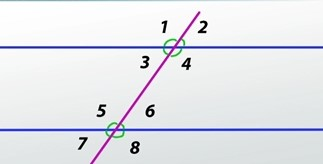A real-life example of a transversal line would be a street cutting across railroad tracks. Since the railroad tracks will remain the same distance apart, the angles will have orderly relationships and many of them will be congruent. The congruent angles are created because of the equal distance between the parallel lines.

### Transversal on Non-Parallel Lines

When a transversal cuts across non-parallel lines, some of the special angles will exist like with parallel lines, but not all of them. The distance between the non-parallel lines will change the angle measurements.

## What Is a Transversal?

A transversal is two parallel lines intersected by a third line at an angle. The third line is referred to as the transversal line. When this line happens, several angles are created. You can use these angles to find the measurements of other angles. When using transversals in geometry you can think about puzzle pieces fitting together.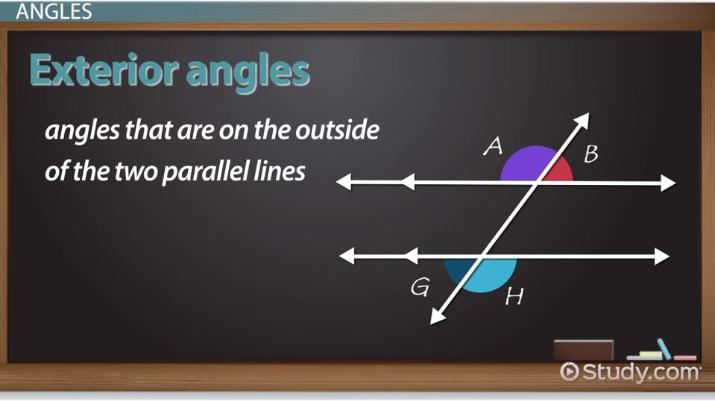An error occurred trying to load this video.

Try refreshing the page, or contact customer support.

Coming up next: What is a Hexagon? - Definition, Area & Angles

### You're on a roll. Keep up the good work!

Replay
Your next lesson will play in 10 seconds
• 0:01 What Is a Transversal?
• 0:23 Angles
• 2:12 Lesson Summary
Save Save

Want to watch this again later?

Timeline
Autoplay
Autoplay
Speed Speed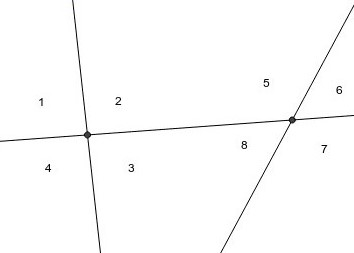## What is a Transversal Angle?

A transversal angle is an angle created when a transversal line cuts across a pair of parallel lines. The transversal creates eight angles with the parallel lines. Let's take a look back at the diagram and break down the relationships between the angles.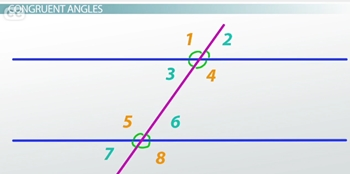The angles can be placed into two groups based on their angle measures. The groups are based on congruency. In the diagram, angles 1, 4, 5, and 8 are congruent. The other group of congruent angles is angles 2, 3, 6, and 7. The two groups will have different measures unless the transversal is perpendicular to the parallel lines. With visual inspection we can see that the angles within each group are congruent.

The angles can be also be grouped based on their location on the transversal and the parallel lines.

• Corresponding Angles are a pair of angles that are on the same side of the transversal, one interior, one exterior, and are congruent. In the diagram the following pairs are corresponding angles: 1 & 5, 2 & 6, 3 & 7, and 4 & 8.
• Consecutive Interior Angles are a pair of angles on the same side of the transversal and between the parallel lines. They are supplementary angles. In the diagram the following pairs are consecutive interior angles: 3 & 5 and 4 & 6.
• Alternate Interior Angles are a pair of non-adjacent angles on the opposite sides of the transversal, between the parallel lines, and are congruent angles. Angles 3 & 6 and 4 & 5 are alternate interior angles.
• Alternate Exterior Angles are a pair of angles on the opposite side of the transversal, outside of the parallel lines, and are congruent angles. The alternate exterior angles are 1 & 8 and 2 & 7.

The transversal cutting across a pair of parallel lines will also create pairs of supplementary angles. These supplementary angles can be found inside and outside of the parallel lines. In the following diagram there are 2 pairs of co-interior angles. The pairs of angles are on the same side of the transversal, inside the parallel lines, and supplementary. In the diagram, angles D & F are a pair and angles C & E are the other pair.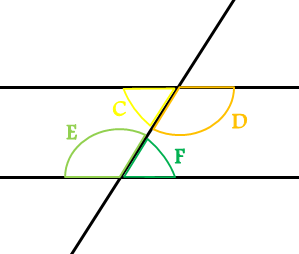We can tell the angles are supplementary because together they make half of a circle, which is {eq}180^\circ {/eq}.

Co-exterior angles are also on the same side of the transversal. They, too, are supplementary but are on the outside of the parallel lines. In the diagram angles B & H are a pair and angles A & G are the other pair of co-exterior angles.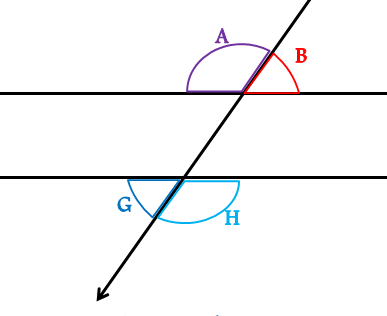## Angles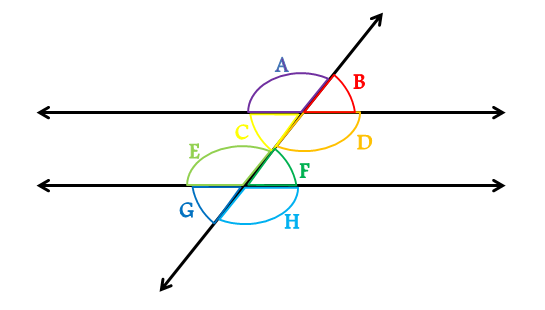There are eight different angles in a transversal. They are placed into five different categories. Knowing these angles can help you solve many geometric problems.

### Supplementary Angles

Supplementary angles are pairs of angles that add up to 180 degrees. If you put two supplementary angle pieces together, you can draw a straight line across the top of the two angles. In essence, the two angles together make a half circle. Supplementary angles are not limited to transversals.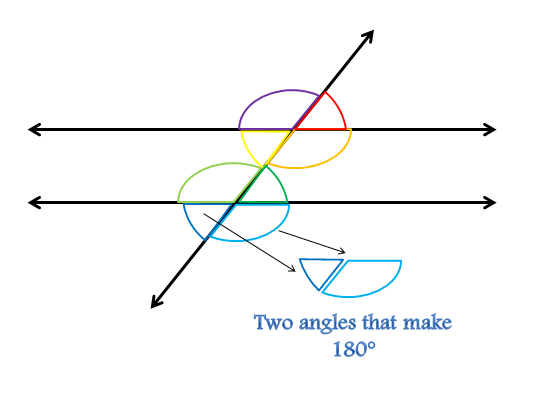In this example, the supplementary angles are AB, CD, EF, GH and AC, BD, EG, FH.

### Interior Angles

Interior angles are angles that are on the inside of the two parallel lines. In the example, the interior angles are angles C, D, E, and F.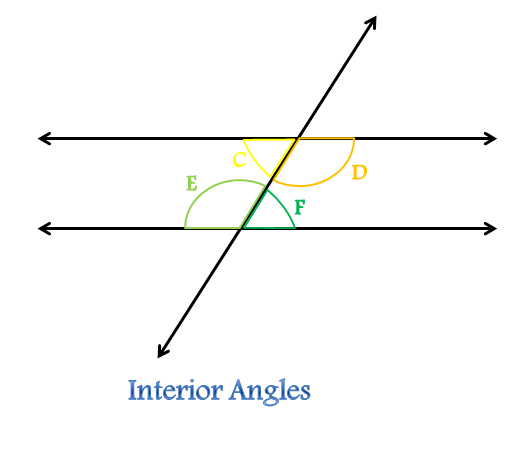### Exterior Angles

Exterior angles are angles that are on the outside of the two parallel lines. In the example, the exterior angles are angles A, B, G, and H.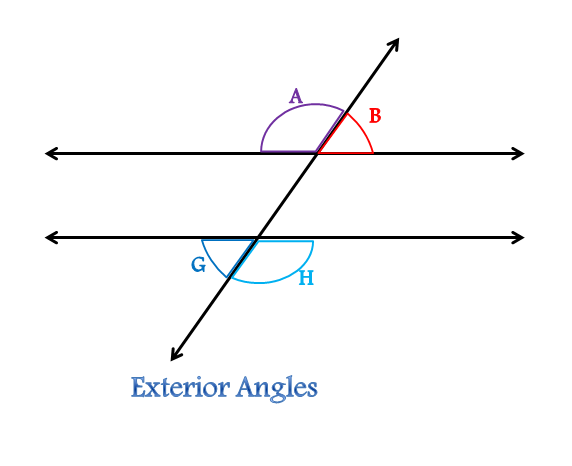### Corresponding Angles

Corresponding angles are two angles that appear on the same side of the transversal line. One of the angles must be an interior angle and the other must be an exterior angle. Corresponding angles are congruent, meaning that they are equal measurements.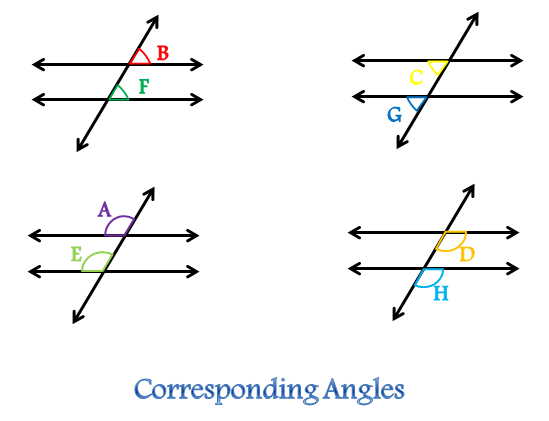To unlock this lesson you must be a Study.com Member.

Video Transcript

## What Is a Transversal?

A transversal is two parallel lines intersected by a third line at an angle. The third line is referred to as the transversal line. When this line happens, several angles are created. You can use these angles to find the measurements of other angles. When using transversals in geometry you can think about puzzle pieces fitting together.

## AnglesThere are eight different angles in a transversal. They are placed into five different categories. Knowing these angles can help you solve many geometric problems.

### Supplementary Angles

Supplementary angles are pairs of angles that add up to 180 degrees. If you put two supplementary angle pieces together, you can draw a straight line across the top of the two angles. In essence, the two angles together make a half circle. Supplementary angles are not limited to transversals.In this example, the supplementary angles are AB, CD, EF, GH and AC, BD, EG, FH.

### Interior Angles

Interior angles are angles that are on the inside of the two parallel lines. In the example, the interior angles are angles C, D, E, and F.### Exterior Angles

Exterior angles are angles that are on the outside of the two parallel lines. In the example, the exterior angles are angles A, B, G, and H.### Corresponding Angles

Corresponding angles are two angles that appear on the same side of the transversal line. One of the angles must be an interior angle and the other must be an exterior angle. Corresponding angles are congruent, meaning that they are equal measurements.To unlock this lesson you must be a Study.com Member.

#### What's the definition of a transversal?

A transversal is a line that cuts across two or more lines in the same plane. The transversal creates very distinct angle relationships when it crosses the other lines.

#### What does transversal mean in angles?

A transversal angle is an angle created when a transversal line cuts across a pair of parallel lines. The transversal creates 8 distinct angles with the parallel lines.

### Register to view this lesson

Are you a student or a teacher?